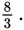# SAT Math Multiple Choice Question 434: Answer and Explanation

### Test Information

Question: 434

14. Use the data in the scatterplot and the line of best fit shown to answer the following question.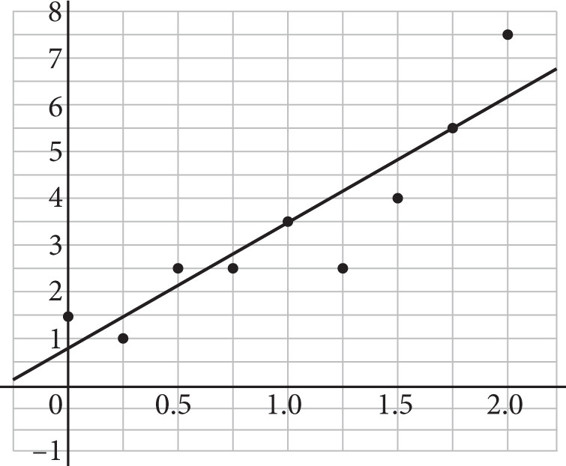Which of the following values most accurately reflects the average rate of change of the data based on the line of best fit?

• A.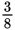• B.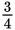• C.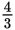• D.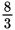Strategic Advice: The average rate of change is the same as the slope of the line of best fit. Look for two points that lie on the line (or as close as possible to the line) and then find the slope using the slope formula,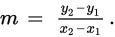Pay careful attention to how the axes are labeled to make sure you write the points accurately.
Getting to the Answer: Using the points (1, 3.5) and (1.75, 5.5), the average rate of change is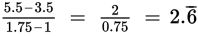or